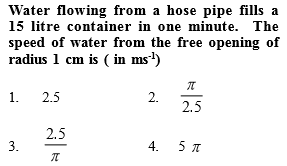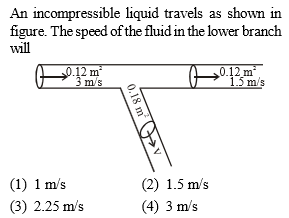#14 | Equation of Continuity
(Physics) > Mechanical Properties of Fluids
Related Practice Questions :Complete Question Bank + Test Series
Complete Question Bank

Difficulty Level:

Equation of continuity based on

1.  Conservation of mass

2.  Conservation of energy

3.  Conservation of angular momentum

4.  None of these

Complete Question Bank + Test Series
Complete Question Bank

Difficulty Level:Complete Question Bank + Test Series
Complete Question Bank

Difficulty Level:

The cylindrical tube of a spray pump has radius R, one end of which has n fine holes, each of radius r. If the speed of the liquid in the tube is v, the speed of the ejection of the liquid through the holes is

(a)vR2/n2r2
(b)vR2/nr2
(c)vR2/n3r2
(d)v2R/nr

Complete Question Bank + Test Series
Complete Question Bank

Difficulty Level:

Two water pipes of diameters 2 cm and 4 cm are connected with the main supply line. The velocity of flow of water in the pipe of 2 cm diameter is

(a) 4 times that in the other pipe

(b) times that in the other pipe

(c) 2 times that in the other pipe

(d) times that in the other pipe